# RD Sharma Class 8 Solutions Chapter 5 Playing With Numbers Ex 5.3

## RD Sharma Class 8 Solutions Chapter 5 Playing With Numbers Ex 5.3

These Solutions are part of RD Sharma Class 8 Solutions. Here we have given RD Sharma Class 8 Solutions Chapter 5 Playing With Numbers Ex 5.3

Other Exercises

Solve each of the following cryptarithms.
Question 1.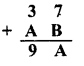Solution: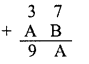Values of A and B be from 0 to 9 In ten’s digit 3 + A = 9
∴ A = 6 or less.
∴ 7 + B = A = 6 or less
∴ 7 + 9 or 8 = 16 or 15
∴ But it is two digit number
B = 8
Then A = 5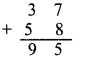Question 2.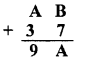Solution:
Values of A and B can be between 0 and 9
In tens digit, A + 3 = 9
∴ A = 9 – 3 = 6 or less than 6
In ones unit B + 7 = A = 6or less
∴ 7 + 9 or 8 = 16 or 15
But it is two digit number
∴ B = 8 and
∴ A = 5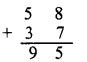Question 3.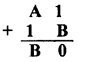Solution: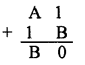Value of A and B can be between 0 and 9 In units place.
1+B = 0 ⇒1+B = 10
∴ B = 10 – 1 = 9
and in tens place
1 + A + 1 = B ⇒ A + 2 = 9
⇒ A = 9 – 2 = 7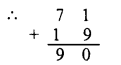Question 4.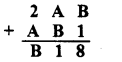Solution:
Values of A and.B can be between 0 and 9
In units place, B+1 = 8 ⇒ B = 8-1=7
In tens place A + B= 1 or A + B = 11
⇒ A + 7 = 11 ⇒ A =11-7 = 4

Question 5.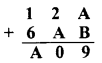Solution: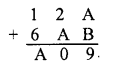Values of A and B can be between 0 and 9
In tens place, 2 + A = 0 or 2 + A=10
A = 10-2 = 8
In units place, A + B = 9
⇒ 8 + B = 9 ⇒ B = 9- 8 = 1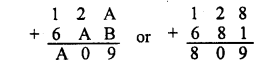Question 6.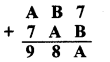Solution: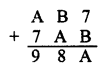Values of A and B can be between 0 and 9
In hundreds place,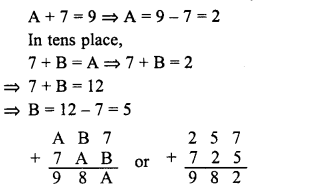Question 7.
Show that cryptarithm 4 x $$\overline { AB } =\overline { CAB }$$ does not have any solution.
Solution: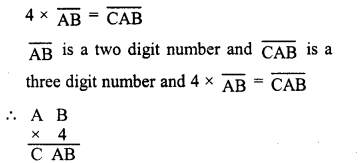It means that 4 x B is a numebr whose units digit is B
Clearly, there is no such digit
Hence the given cryptarithm has no solution.

Hope given RD Sharma Class 8 Solutions Chapter 5 Playing With Numbers Ex 5.3 are helpful to complete your math homework.

If you have any doubts, please comment below. Learn Insta try to provide online math tutoring for you.